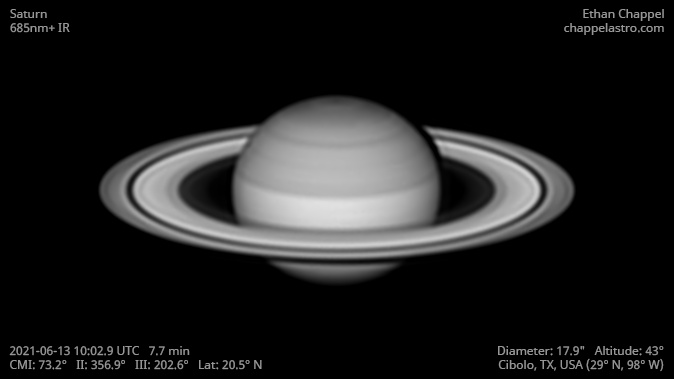# Saturn 2021-06-13 10:02 UTC

CM1: 73.20°

CM2: 356.90°

CM3: 202.60°

CLat: +20.50°

Description

Excellent seeing conditions. This is a second attempt with my EdgeHD 11 and new Sky-Watcher EQ6-R Pro after a near-failure yesterday because of clouds. My primary use for this mount will be for deep sky imaging, but I can also use it for planetary imaging with the EdgeHD 11 in times I would've used the EdgeHD 8 and Advanced VX.

Notes:

• The perimeter of the North Polar Hexagon is bright in near-infrared, making it stand out more than in visible light.

Equipment

ZWO ASI290MM

Celestron EdgeHD 11

Sky-Watcher EQ6-R Pro

Logs
```FireCapture v2.7 04 BETA Settings
------------------------------------
Observer=Ethan Chappel
Location=Cibolo
Scope=Celestron C8 EdgeHD
Camera=ZWO ASI290MM
Filter=IR685
Profile=Saturn
Diameter=17.82"
Magnitude=0.44
CMI=74.6° CMIII=204.0°  (during mid of capture)
FocalLength=4550mm (F/22)
Resolution=0.13"
Filename=s2021-06-13_10-05-29_ir685_ec.ser
Date=2021_06_13
Start=10_04_14.469
Mid=10_05_29.471
End=10_06_44.473
Start(UT)=10_04_14.469
Mid(UT)=10_05_29.471
End(UT)=10_06_44.473
Duration=150.004s
Date_format=yyyy_MM_dd
Time_format=HH_mm_ss
LT=UT
Frames captured=14986
File type=SER
Binning=1x1
ROI=300x430
ROI(Offset)=1256x328
FPS (avg.)=99
Shutter=10.00ms
Gain=440 (73%)
AutoExposure=off
AutoGain=off
Brightness=1
HighSpeed=on
USBTraffic=50
Histogramm(min)=0
Histogramm(max)=203
Histogramm=79%
Noise(avg.deviation)=1.94
Limit=150 Seconds
Sensor temperature=95.4°F
FireCapture v2.7 04 BETA Settings
------------------------------------
Observer=Ethan Chappel
Location=Cibolo
Scope=Celestron C8 EdgeHD
Camera=ZWO ASI290MM
Filter=IR685
Profile=Saturn
Diameter=17.82"
Magnitude=0.44
CMI=73.1° CMIII=202.6°  (during mid of capture)
FocalLength=4550mm (F/22)
Resolution=0.13"
Filename=s2021-06-13_10-02-57_ir685_ec.ser
Date=2021_06_13
Start=10_01_42.219
Mid=10_02_57.221
End=10_04_12.223
Start(UT)=10_01_42.219
Mid(UT)=10_02_57.221
End(UT)=10_04_12.223
Duration=150.004s
Date_format=yyyy_MM_dd
Time_format=HH_mm_ss
LT=UT
Frames captured=14986
File type=SER
Binning=1x1
ROI=300x430
ROI(Offset)=1256x328
FPS (avg.)=99
Shutter=10.00ms
Gain=440 (73%)
AutoExposure=off
AutoGain=off
Brightness=1
HighSpeed=on
USBTraffic=50
Histogramm(min)=0
Histogramm(max)=214
Histogramm=83%
Noise(avg.deviation)=1.95
Limit=150 Seconds
Sensor temperature=95.4°F
FireCapture v2.7 04 BETA Settings
------------------------------------
Observer=Ethan Chappel
Location=Cibolo
Scope=Celestron C8 EdgeHD
Camera=ZWO ASI290MM
Filter=IR685
Profile=Saturn
Diameter=17.82"
Magnitude=0.44
CMI=71.6° CMIII=201.0°  (during mid of capture)
FocalLength=4550mm (F/22)
Resolution=0.13"
Filename=s2021-06-13_10-00-15_ir685_ec.ser
Date=2021_06_13
Start=09_59_00.782
Mid=10_00_15.784
End=10_01_30.786
Start(UT)=09_59_00.782
Mid(UT)=10_00_15.784
End(UT)=10_01_30.786
Duration=150.004s
Date_format=yyyy_MM_dd
Time_format=HH_mm_ss
LT=UT
Frames captured=14986
File type=SER
Binning=1x1
ROI=300x430
ROI(Offset)=1256x328
FPS (avg.)=99
Shutter=10.00ms
Gain=440 (73%)
AutoExposure=off
AutoGain=off
Brightness=1
HighSpeed=on
USBTraffic=50
Histogramm(min)=0
Histogramm(max)=225
Histogramm=88%
Noise(avg.deviation)=1.95
Limit=150 Seconds
Sensor temperature=95.0°F
```